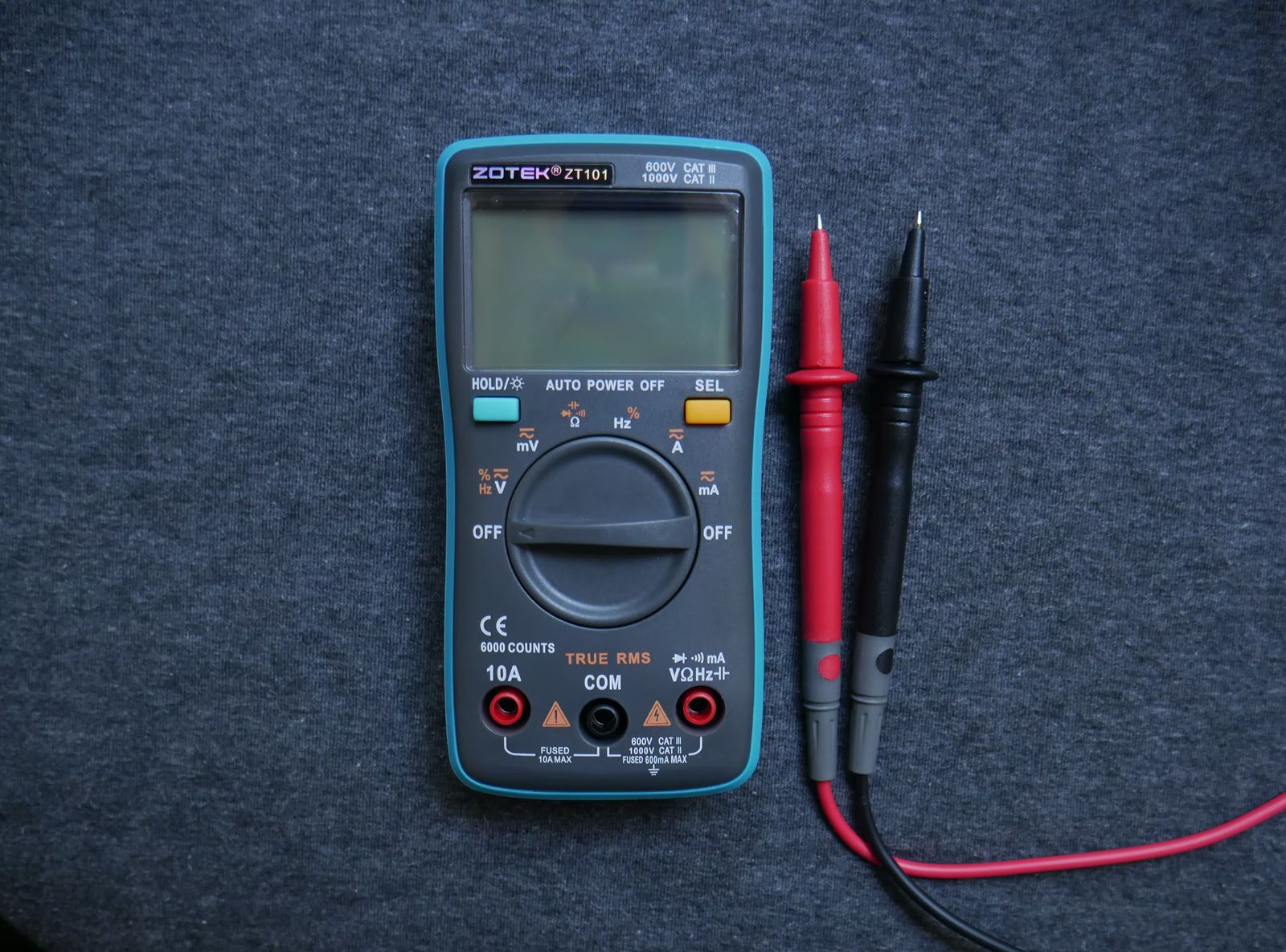## Capacitance Units

What are the base SI units for capacitance?Hint
Capacitance is the ratio of the amount of electric charge stored on a conductor to a difference in electric potential. The units for capacitance is the farad (F).
Hint 2
The units for capacitance is the farad (F), which is 1 coulomb of electrical charge over 1 volt of potential difference.
$$1\:F=\frac{1\:Coulomb}{1\:Volt}$$$Capacitance is the ratio of the amount of electric charge stored on a conductor to a difference in electric potential. The units for capacitance is the farad (F), which is 1 coulomb of electrical charge over 1 volt of potential difference. $$1\:F=\frac{1\:Coulomb}{1\:Volt}$$$
The coulomb is the unit for electrical charge. It is defined as an ampere x second.
$$1\:Coulomb=1\:Ampere\cdot 1\:Second$$$Voltage is the electrical potential difference between two points, which is defined as the work (watts) needed per unit of charge (ampere). $$1\:V =1\:\frac{W}{A}$$$
Power is the time rate of doing work. The unit for power is a watt (W), which is one joule per second.
$$1\:W=1\:\frac{J}{s}$$$Joule (J) is a unit of work, which is the product of a unit force (one newton) acting through a unit distance (one meter). $$1\:J=1\:N\cdot m$$$
A newton is a unit of force, which is described in the following SI base units:
$$\frac{m\cdot kg}{s^{2}}$$$Combining all units together: $$F=\frac{C}{V}=\frac{A^{2}\cdot s}{W}=\frac{A^{2}\cdot s^{2}}{J}=\frac{A^{2}\cdot s^{2}}{N\cdot m}=\frac{A^{2}\cdot s^{4}}{kg\cdot m^2}$$$
$$\frac{A^{2}\cdot s^{4}}{kg\cdot m^2}$$\$